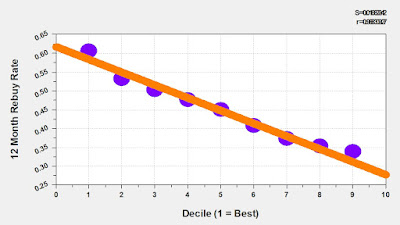## May 15, 2018

### New Items, Demand Growth

Here's six years of new merchandise introductions, and the demand generated by the new merchandise introductions. That's the top of the table ... the bottom of the table converts the numbers to an index based on 2017 results.

Ok, let's plot the relationship between each index.

Oh - that's the a*(x^b) relationship!
• a = 0.994.
• b = 0.394.
With the relationship we can calculate how much demand we generate when we vary new item introductions ... cut back by 80% and we lose 55% of demand ... increase by 40% and we increase demand from new items by 16%.

Now we can plan what an appropriate merchandise assortment might be.

Fun!